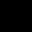# P, Q, R, S, T and U are six cities which are in a row in the same order. A bus, b1 travels from P to U and another bus b2 travel from U to P. The bus b1 reaches S at 10:40 and bus b2 reaches Q at 10:35 if the travel time between any two adjacent cities is 40 minutes and the stoppage time at each city is 15 minutes. Which city do the two buses meet?

## 3 Answers on this Question

1.Anu says:

An

2.THANATI says:

Hi

3.DK BOSS says:

Explanation:

The bus b1, which started at P, reached S at 10:40, passing through the intermediary cities Q and R.

The time taken to travel from P to S
= 3 * 40 + 2 * 15 = 150minute
(journey)+(stoppage) = 2 hrs 30 minutes.
Hence, b1 started at 10:40 – 2:30 = 8:10 at P.
b2 reached Q, starting at U, through the city T, S and R.
The time taken by it to reach S = 4 * 40 + 3 * 15 = 205 minutes = 3 hr 25 minutes.
Hence, b2 started at, 10:35 – 3:25 = 7:10, at U.

Scroll to top